WPS Office

Free All-in-One Office Suite with PDF Editor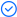Read, edit, and convert PDFs with the powerful PDF toolkit.Microsoft-like interface, easy to use.

Windows • MacOS • Linux • iOS • Android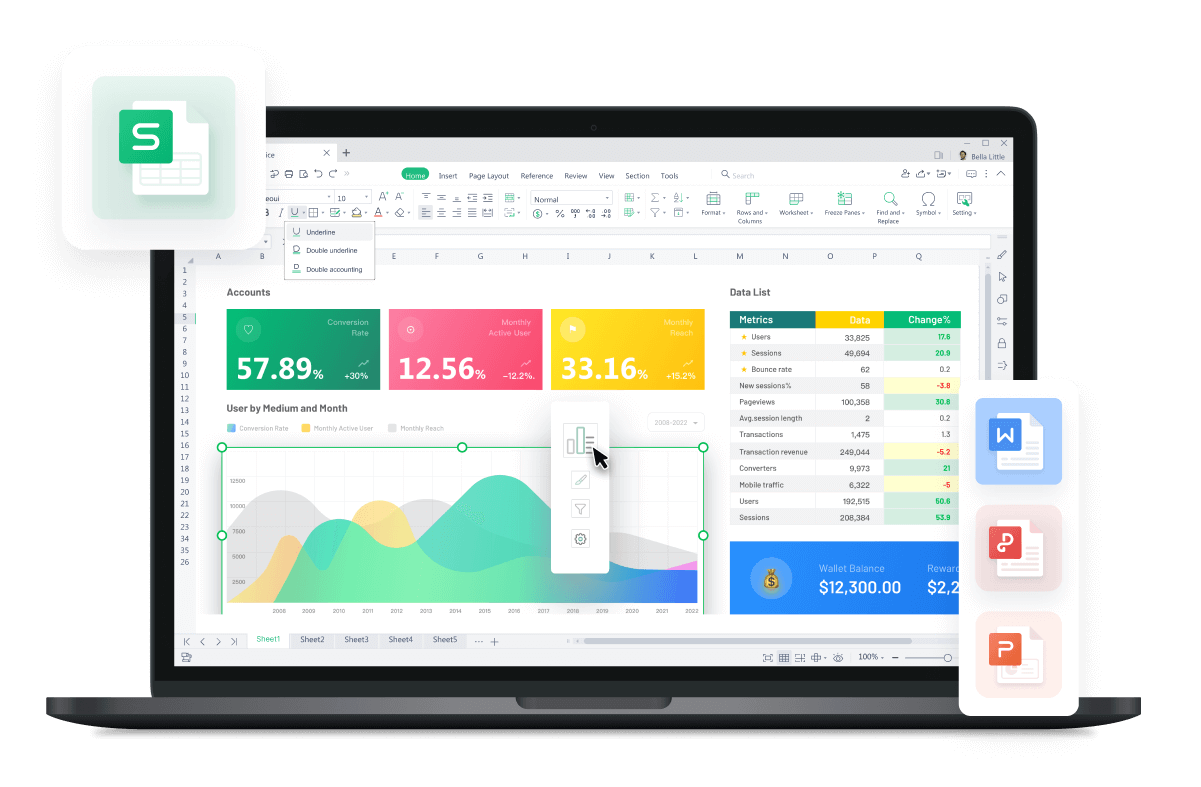# How to Use Sum Function in Excel

July 26, 2022
7.9K Views

SUM Function in Excel is a part of math function. It can be used as a worksheet function in Excel and this function is used to count the number of cells that contain numbers. If a cell is empty or not numeric, it will be ignored. This article will explain how to use sum function in excel.

If you know how to use sum function in excel, the excel SUM function can be used to quickly add up the values contained in a range of cells. It falls within the Math and Trigonometry category of functions, and its input consists of typing =SUM followed by the values that are to be added up at the end. The function can take in values in the form of numbers, cell references, or range references as its parameters.

## Definition of SUM Function

The SUM function in Excel is a built-in mathematical function that calculates the sum of a range of cells. It adds up the numerical values in the specified range and returns the total. The SUM function is commonly used to quickly calculate the total of a column or row of numbers. It can also be used with multiple ranges or as part of more complex formulas for advanced calculations.

## Getting the Sum of a Column Using SUM Function

### Steps

Step 1 Click the cell in your table where you want to view the total of the cells you've selected.

Step 2 Insert the SUM function into the chosen cell.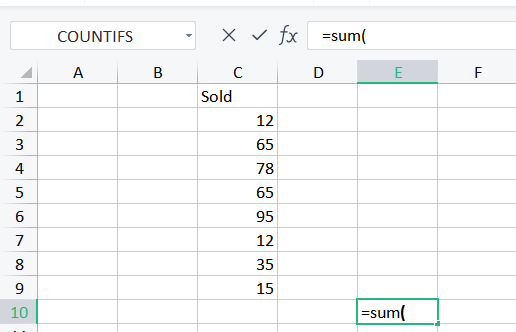Step 3 Now, pick the range of numbers to total and press Enter on your keyboard.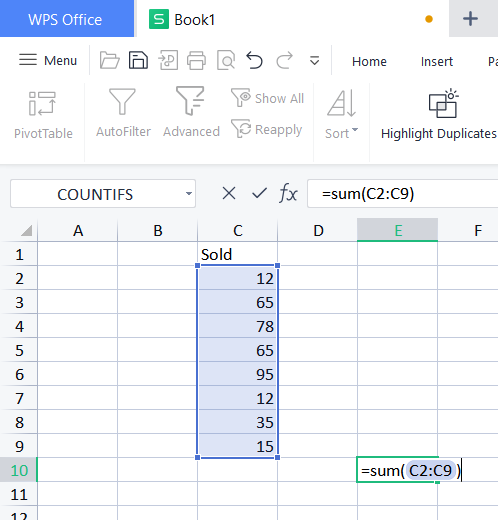Tip: You can manually input the range address, such as =sum(B1:B2000). It comes in handy when working with vast ranges.

That's all! The column will be totaled. The sum will be displayed in the appropriate cell.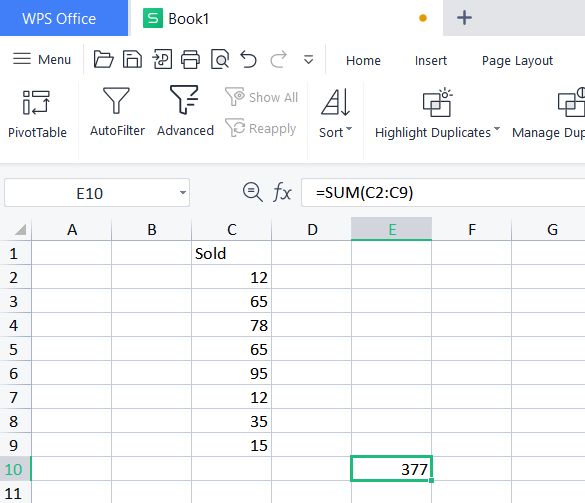## Troubleshooting Common Errors in Excel SUM Function

Excel formulas not working:

• Check for proper pairing of parentheses and ensure all required arguments are included.

• Avoid nesting more than the allowed number of functions in a formula.

• Remove double quotes around numbers to prevent them from being treated as text.

• Enter numbers without formatting or symbols like commas or currency signs.

• Make sure numbers are not formatted as text values.

• Separate function arguments with the appropriate character, based on regional settings.

• Enclose workbook and worksheet names in single quotes if they contain spaces or special characters.

• Include the full path to a closed workbook if referencing it in a formula.

Excel formulas not updating:

• Check if the Calculation setting is set to Manual. Change it to Automatic for automatic updates.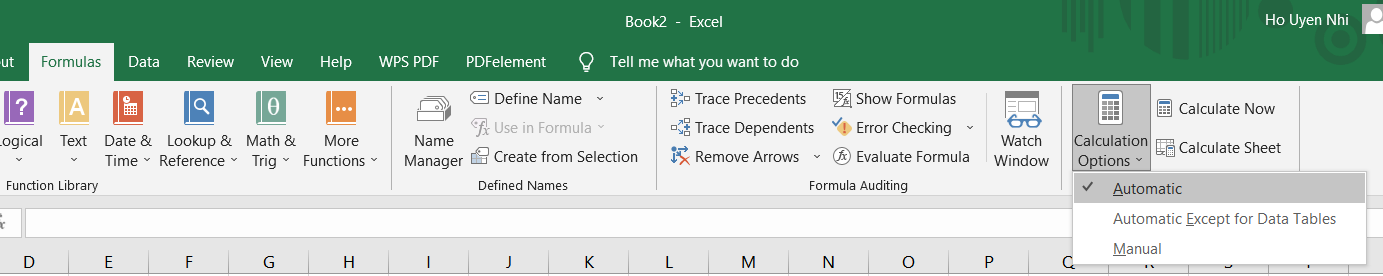• To force recalculation, use the Calculate button or shortcut keys based on the desired scope (entire workbook, active sheet, or all sheets).

Press F9 or the Calculate Now button on the Formulas tab > Calculation Now group. ( entire work book)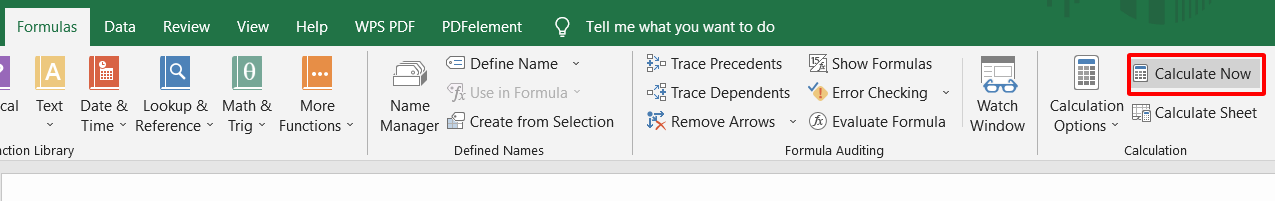Shift + F9, or Calculate Sheet in the Formulas tab > Calculation group. ( active sheet)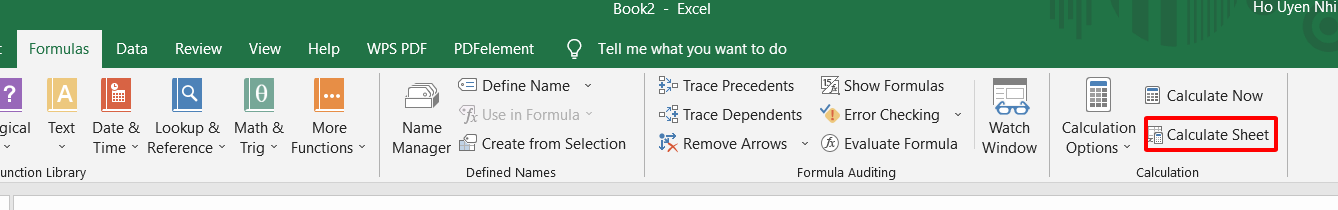Excel formulas not calculating:

• Ensure the Show Formulas mode is turned off.

• Verify that the formula is not formatted as text.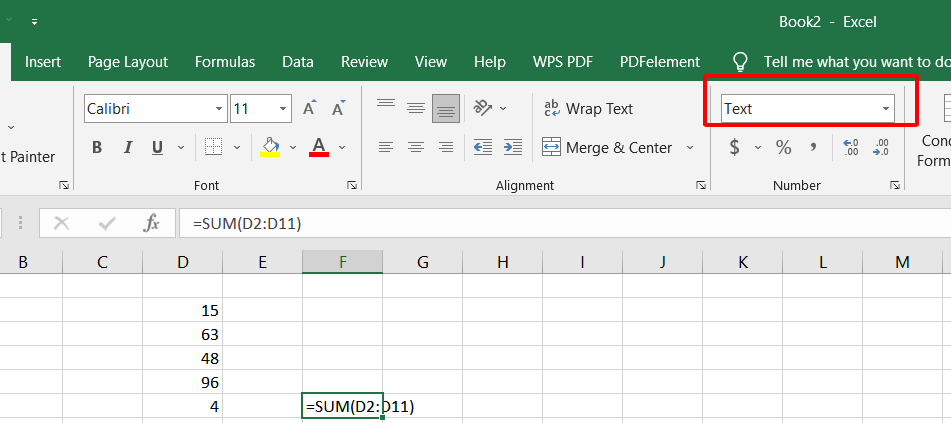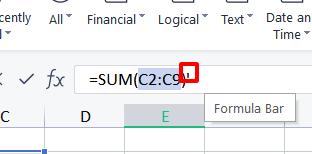## Extending SUM: The SUMIF and SUMIFS Functions

### SUMIF Function:

The SUMIF function allows you to sum values based on a single condition. It takes three arguments: range, criteria, and sum_range. The function evaluates each cell in the range, and if the corresponding cell meets the specified criteria, it adds the corresponding value from the sum_range.

### SUMIFS Function:

The SUMIFS function expands on the capabilities of SUMIF by allowing you to sum values based on multiple conditions. It takes pairs of arguments: range/criteria pairs. The function evaluates each cell in the specified ranges, and if all the corresponding cells meet their respective criteria, it adds the values from the sum_range.

## Excel Alternatives for Getting Column Sum

### Using Excel Pivot Tables for Summation

Step 1 Right-click the value field you wish to update in the PivotTable, and then select Summarize Values By.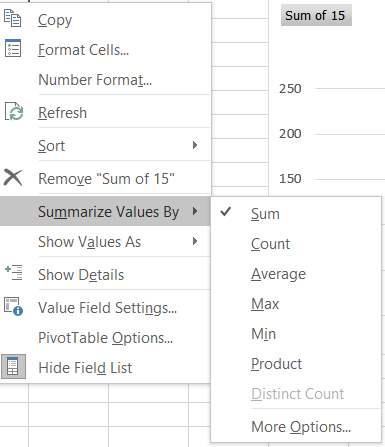Step 2 Select the desired summary function by clicking it.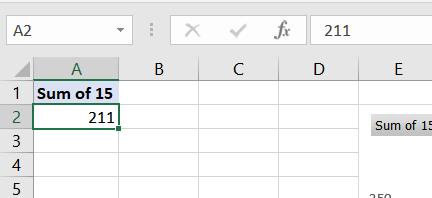### Exploring the SUBTOTAL Function

As its initial parameter, the SUBTOTAL function always takes a number input (1 through 11, 101 through 111). This number parameter is applied to the subtotal of the values (cell ranges, named ranges) supplied in the following arguments.

For example

The sum of the subtotal of the cells C2:C10, using 9 as the first argument.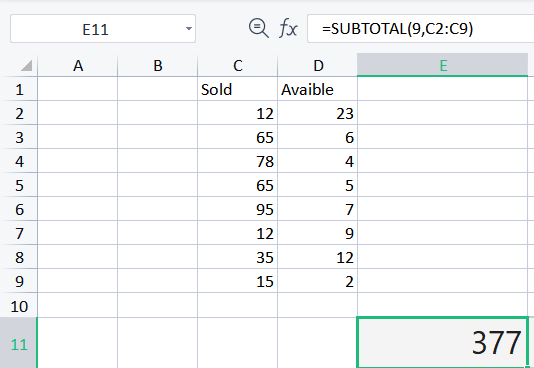The average of the subtotal of the cells C2:C10, using 1 as the first argument.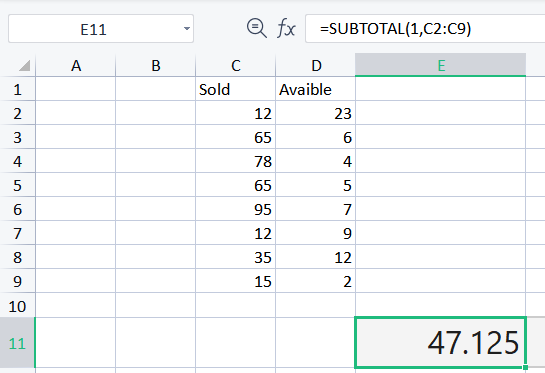WPS Spreadsheet, commonly known as WPS Excel, is a component of the WPS Office suite. WPS Office is a comprehensive office productivity suite that includes word processing, presentation, and spreadsheet applications.

WPS Spreadsheet offers a range of features and functions similar to Microsoft Excel, allowing users to create, edit, and analyze data in a spreadsheet format. With its user-friendly interface and compatibility with Excel file formats, WPS Spreadsheet provides a viable alternative for spreadsheet tasks and data management needs.

Trustpilot4.8
WPS Office- Free All-in-One Office Suite

• Edit PDF files with the powerful PDF toolkit.

• Microsoft-like interface. Easy to learn. 100% Compatibility.

• Boost your productivity with WPS's abundant free Word, Excel, PPT, and CV templates.

5,820,008 UserAlgirdas Jasaitis

## FAQs

### What are Some Alternatives to the SUM function for Getting Column Sums?

Apart from the SUM function, you can use other functions like SUMPRODUCT, SUBTOTAL, and AGGREGATE to calculate column sums in Excel. These functions offer additional functionalities and can be useful in specific scenarios.

### How do I Correct a #Value Errors in My SUM Function Formula?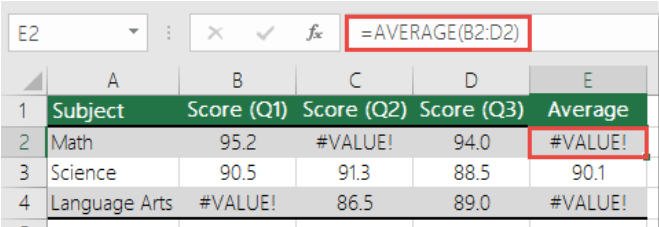To avoid this problem, we utilize AVERAGE in conjunction with IF and ISERROR to detect if there is an error in the provided range. This circumstance necessitates the use of an array formula:

=AVERAGE(IF(ISERROR(B2:D2),"",B2:D2))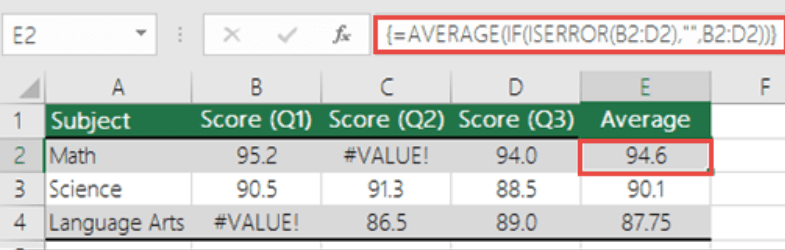### What is One advantage of Using SUM Function in Excel?

One advantage of using the SUM function in Excel is its ability to calculate totals for both contiguous and non-contiguous ranges of cells. This means that you can easily obtain the sum of values even if they are scattered across different parts of your worksheet.

### How to Use AutoSum to Get Column Sum?

To use AutoSum and quickly get the sum of a column in Excel, follow these steps:

Step 1 Place your cursor in the cell directly below the column of numbers you want to sum.

Step 2 Press Alt + = on your keyboard. This keyboard shortcut automatically selects the range of cells above the current cell and inserts the SUM formula.

Step 3 Press Enter to complete the AutoSum. The sum of the column will be calculated and displayed in the current cell.

## Conclusion

This article provides an explanation of the features that are associated with how to use Sum Function in Excel to get the correct and quick result. If, on the other hand, you are interested in learning more about the WPS office, I would like to direct your attention to the official website of the WPS Academy. The free version of WPS Office, which can be downloaded from this website, enables users to make changes to a variety of file types, including PDFs, Word spreadsheets, and PowerPoint presentations.